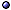Abstract Algebra

In General > s.a. elementary (real and complex) algebra; Homological Algebra.
* Idea: The study of properties of sets A with some operations, internal or external (defined with a field K).
@ General references: Van der Waerden 31; Bourbaki 4262; Jacobson 5164; Birkhoff & MacLane 53; Chevalley 56; Kurosh 65; Mac Lane & Birkhoff 67; Goldhaber & Ehrlich 70; Kurosh 72; Lang 84; Fan et al 99; Hazewinkel et al 04 [+ rings and modules]; Knapp 06; Eie & Chang 10; Adhikari & Adhikari 14 [IIb]; Reis & Rankin 16; Lawrence & Zorzitto 21 [intro].

Algebra > s.a. Character; Coalgebra; Division Algebra; Ideal; ring.
$Def: A vector space (V, +, K) with a multiplication · such that (V, +, · ) is a ring, and α(xy) = (αx) y = x(αy) for all αK and x, yV. * Result: There are about 1151 consistent algebras in addition to the reals, which can be formulated by weakening the Field axioms (more than 200 have been rigorously proven to be self-consistent). @ Operations: Borowiec & Marcinek JMP(00) [crossed product]. > Examples: see quaternions. Banach Algebra$ Def: A complete normed algebra.
* Result: (Gel'fand) If every non-zero a in a commutative Banach algebra with identity A is invertible, A is isomorphic to $$\mathbb C$$.
* Result: If A is a commutative Banach algebra with identity, and C a maximal ideal, then A/C ≅ $$\mathbb C$$; Thus, there is a correspondence between maximal ideals C and kernels of characters χ; ker χ = C.

Commutative Algebra
@ General references: Zariski & Samuel 58, 60; Bourbaki 62; Hartley & Hawkes 70; Stanley 83; Kunz 85; Li 04; Patil & Storch 10 [and algebraic geometry]; Singh 11.
@ Differential calculus: Baehr et al JPA(95).

*-Algebra > s.a. C*-algebra.
\$ Def: An algebra $$\cal A$$ with an involution operation *: $$\cal A$$ → $$\cal A$$.
* Involution: A map *: $$\cal A$$ → $$\cal A$$ on an algebra over K, which is anti-linear, (A + λB)* = A* + λ* B*, where * is complex conjugation if K = $$\mathbb C$$ and has no effect if K = $$\mathbb R$$, and satisfies (AB)* = B*A*, (A*)* = A.
@ References: Bagarello JMP(08) [O*-algebras and quantum dynamics].

Other Algebras and Generalizations > s.a. deformation quantization; observable algebras.
* Moyal star-product deformation: If $$\cal A$$ is the algebra C($$\mathbb R$$2) or the real/complex polynomials, we can define the deformed associative product

$(F \star_\hbar G)(p,q):= FG(p,q) + \sum_{k\ge1} {({\rm i}\hbar)^k\over2^k k!}\,\{F,G\}_k(q,p) \;,$

${\rm where}\quad \{F,G\}_k:= \sum_{i=0}^k (-1)^i {k\choose i}\, {\partial^kF\over\partial^{k-i}p\,\partial^iq}\,{\partial^kG\over\partial^ip\,\partial^{k-i}q}\;.$

This is the unique non-trivial deformation of $$\cal A$$; Notice that {F,G}1 is the Poisson bracket.
* Filtered algebra: One that that be written as A = ∪k = 0 A(k), with A(k)A(k+1); e.g., observable algebras.
* Malcev algebra: A (non-associative) algebra in which the product satisfies x2 = 0 for all x and J(x, y, xz) = J(x, y, z) x for all x, y, z, where J(x, y, z):= (xy) z + (yz) x + (zx) y [the Jacobi-type combination of three elements]; Example: All Lie algebras, in which J(x, y, z) = 0 for all x, y, z.
* Topological algebra: An algebra with a suitably related (quasi)topological structure; The theory was developed in the late 1930s by Gel'fand and others; Examples are function, operator, and Banach algebras.
@ Topological: Beckenstein, Narici & Suffel 77.
@ Bialgebras: Xu m.QA/00-conf [Gel'fand-Dorfman, rev]; Rezaei-Aghdam et al a1401 [Leibniz bialgebras]; > s.a. lie algebras.
@ n-ary algebras: Goze et al JAPM-a0909; Fairlie & Nuyts JPA(10)-a1007 [conditions for ternary algebras].
@ Related topics: in Jordan in(72) [Malcev]; Jaganathan mp/00 [quantum, intro]; Bandelloni & Lazzarini NPB(01)ht/00 [W3]; Gudder & Greechie IJTP(05) [sequential effect algebras]; Doubek et al a0705-ln [deformation theory]; Huang et al IJTP(10) [causal algebras].Examples: see Boolean, clifford, Cluster, Cuntz, Fréchet, Galois, grassmann, Hecke, Heyting, Hopf, lie, poisson algebra; deformation quantization [Moyal algebra]; [Sigma-Algebra]; Simple Algebra; Tangle [diassociative algebras]; Temperley-Lieb Algebra.

Online Resources > see Internet Encyclopedia of Science pages.